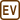## Free and Displacement Current in Water

Consider a plate capacitor immersed deep in seawater with its plates close together; time-varying voltage applied across the plates is

$u = U_0\,\cos\,(2\pi ft), \qquad {\rm where} \ \ f = 4 {\cdot} 10^{8}\ {\rm Hz}.$

At this frequency, the permittivity of seawater is approximately ε = 81ε0, permeability is approximately μ = μ0 and electric resistivity is ρ = 0.23 Ω·m.

Estimate the amplitude ratio of a density of free current and a density of Maxwell’s displacement current between the plates using quasi-stationary approach. Consider whether the use of quasi-stationary approach is correct.

• #### Hint – useful relations

What is the relation between

• the voltage and the electric field intensity between the plates (in quasi-stationary approach),
• the density of free current and the electric field intensity,
• the density of displacement current and the electric displacement field.
• #### Analysis

In the plate capacitor we can – considering the quasi-stationary approach, in which the laws of electrostatics apply – calculate the amplitude of the electric intensity depending on the voltage between the electrodes.

Knowing the behaviour of the electric intensity function, we can calculate the density of displacement current by using Ohm’s law (in differential form) and the density of displacement current definition (partial derivatives of electrical displacement $$\vec{D}$$ by time). Then we simply compare their amplitudes.

It is certainly a question of how much is quasi-stationary approach correct at almost GHz voltage frequency. A simple criterion says that it can be safely used if the amplitude of the displacement current is much smaller than the amplitude of the free current.

• #### Solution

The size of homogeneous electric field intensity E can be, when applying the quasi-stationary approach, evaluated as

$E = \frac{u}{d} = \frac{U_0}{d}\,\cos\,(2\pi ft),$

where d is the distance of the plates. According to Ohm’s law in differential form the size of the density of free current between the plates is

$j = \sigma E = \frac{1}{\varrho}\frac{u}{d} = \frac{U_0\cos(2\pi ft)}{\varrho d}.$

When adjusting, we used the relationship between the specific electrical conductivity σ and the electrical resistivity ρ = 1/σ. The size of the density of displacement current between the plates is then

$j_d = \frac{\partial D}{\partial t} = \varepsilon\frac{\partial E}{\partial t} = -\frac{\varepsilon}{d}U_0\,\cdot\, 2\pi f\, \cdot\, \sin(2\pi ft).$

The ratio of amplitudes of the density of free current and displacement current is therefore

$\frac{j_{max}}{j_{d,max}} = \frac{\frac{U_0}{\varrho d}}{2\pi f U_0\frac{\varepsilon}{d}} = \frac{1}{2\pi f\varepsilon\varrho}\, \dot{=}\, 2.4.$

Free current and displacement current are, under these conditions, of the same order and of comparable size. The use of quasi-stationary approach in this case is not entirely correct and the calculation must be taken more as an estimation, or as a simple check of the (in)validity of the quasi-stationary approach.

$\frac{j_{max}}{j_{d,max}} \dot{=}\, 2.4,$